# Congruence equation

(diff) ← Older revision | Latest revision (diff) | Newer revision → (diff)

algebraic congruence

A congruence of the form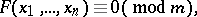(1)

where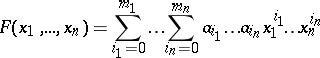is a polynomial in the variableswith integral rational coefficients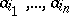andis an integer. The maximum value of the magnitudewhere the maximum is taken over all possible tuplesfor which(), is called the degree with respect to the set of variablesor the degree of (1). The maximum value of the magnitudes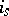,, where the maximum is taken over the same tuples, is called the degree of the congruence equation with respect to the variable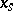.

The principal problem in the theory of congruence equations is the number of solutions of a given congruence. It is possible to restrict the problem to the case of a prime module, since the problem of the number of solutions of (1) by a composite module, except for a few degenerate cases, may be reduced to the problem of the number of solutions of the congruences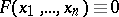(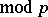) for the prime modulesthat are divisors of.

The most thoroughly studied congruence equations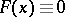() in one variable are the two-term congruences (cf. Two-term congruence)The study of the number of solutions of congruences in the case of a general polynomialis very difficult, and only isolated partial solutions have so far been obtained.

A system of congruences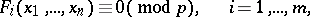(2)

may be considered as a system of algebraic equations:over the finite prime field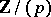consisting ofelements; the number of solutions of this system of congruences will be equal to the number of-rational points of the algebraic variety defined by the system of equations (2). Accordingly, together with number-theoretical methods, the methods of algebraic geometry are also used in the study of congruence equations or systems of such congruences.

The most extensively studied congruence equations in several variables were those of the form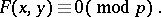The estimate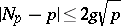(3)

was obtained for the number of solutionsof a congruence of this type, where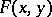is an absolutely irreducible polynomial. The constantdepends only on the polynomial and is equal to the genus of the curve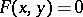. Such an estimate for the first non-trivial case, viz. for the elliptic congruence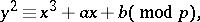was obtained by H. Hasse in 1934, who based his work on the formula for the addition of points on the Jacobi variety of the curve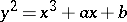. Hasse's method was subsequently extended by A. Weil  to the case of absolutely irreducible polynomials. This estimate was also obtained in  by elementary methods.

Studies of congruence equations with a number of variableshave been much less extensive. One general result is the theorem of Chevalley, according to which ifis a form the degree of which is strictly smaller than the number of variables, then the number of solutions of the congruence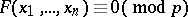is positive and is divisible by; in the case of non-homogeneous polynomials the existence of a solution is not guaranteed, but the divisibility byremains valid (Warning's theorem). This last theorem has been generalized to systems of congruences.

The theory of congruence equations has numerous applications in other branches of number theory — the theory of Diophantine equations, problems in additive number theory, algebraic number theory, etc.

How to Cite This Entry:
Congruence equation. Encyclopedia of Mathematics. URL: http://encyclopediaofmath.org/index.php?title=Congruence_equation&oldid=12343
This article was adapted from an original article by S.A. Stepanov (originator), which appeared in Encyclopedia of Mathematics - ISBN 1402006098. See original article